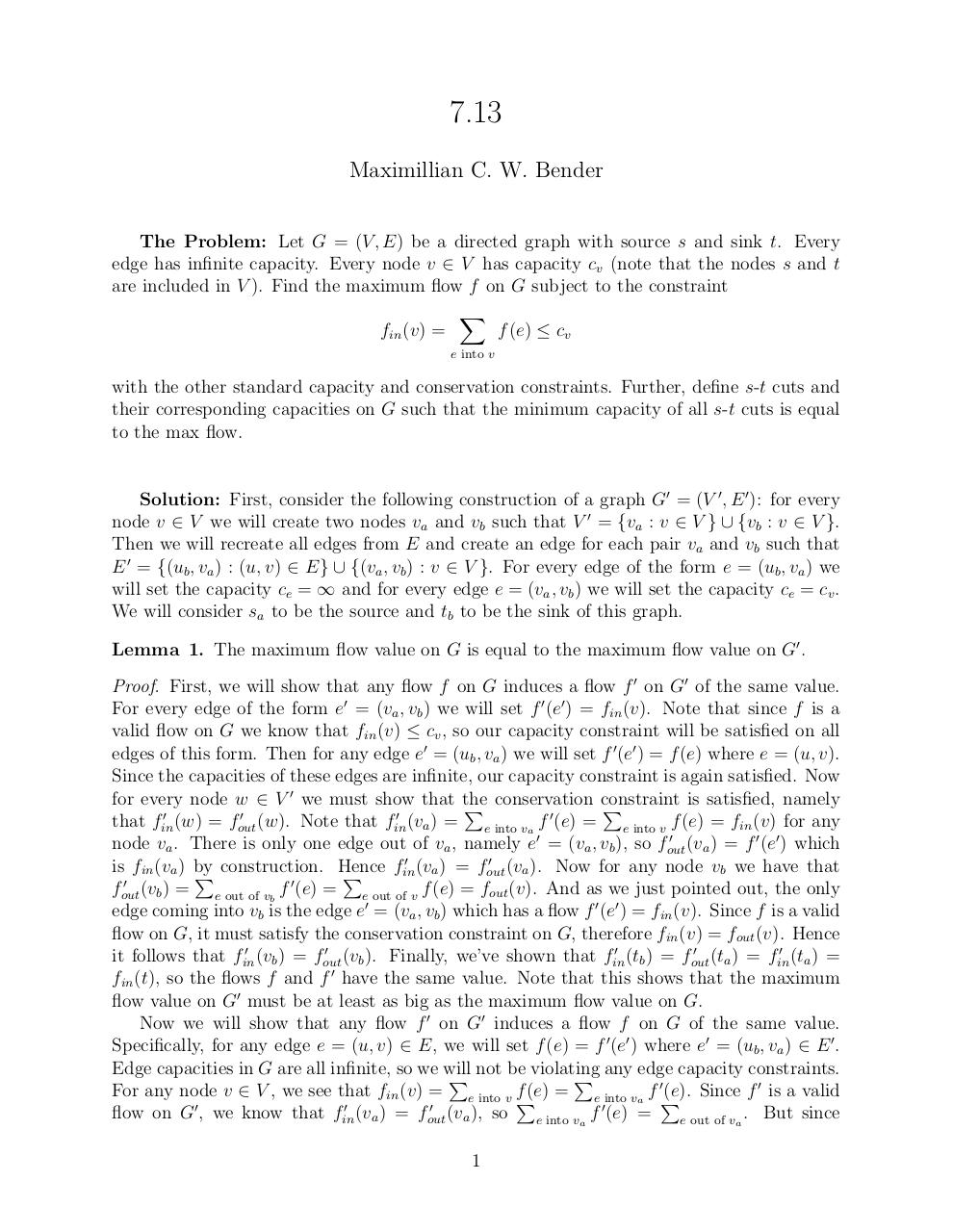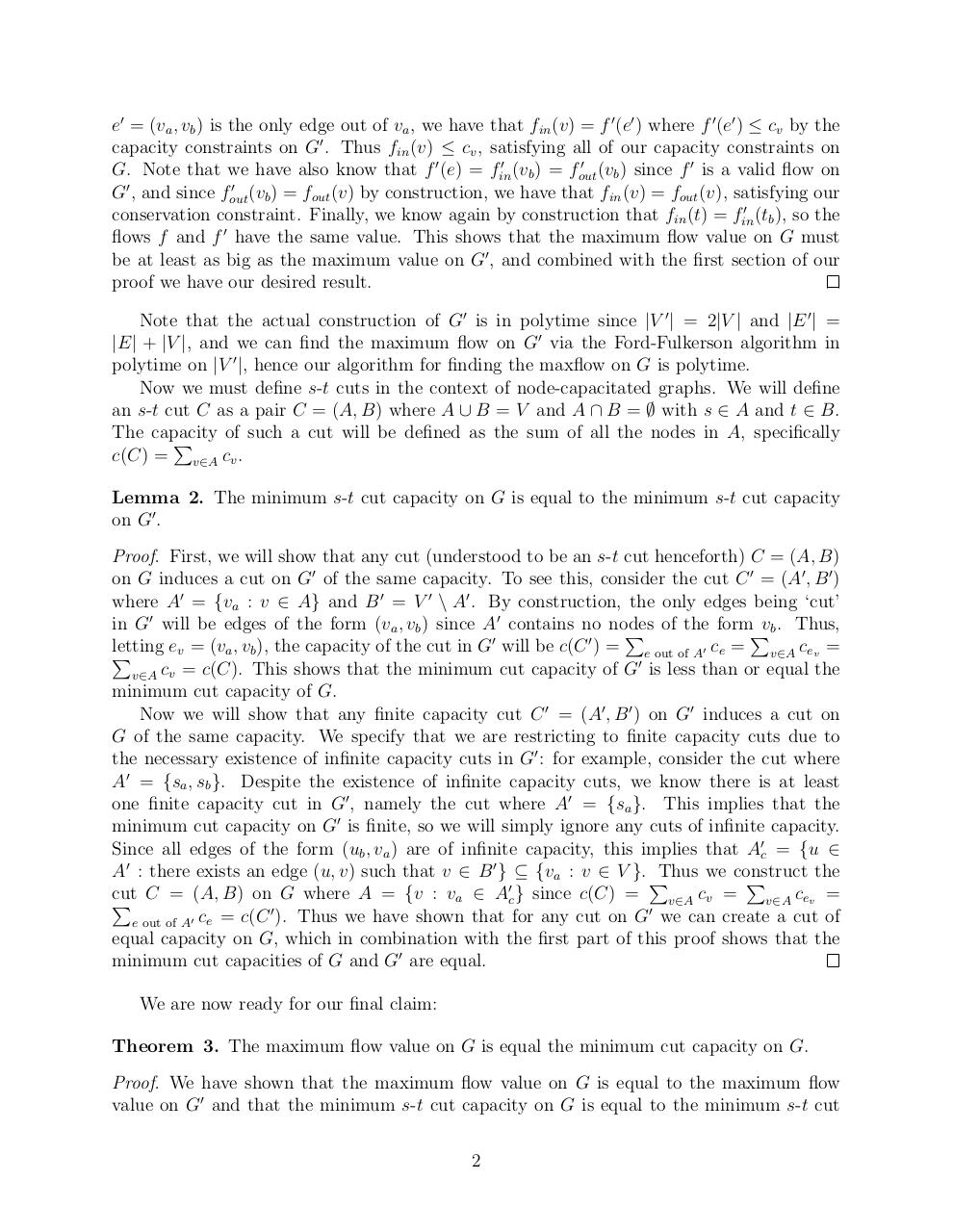# Algorithm7 13 .pdf

### File information

Original filename: Algorithm7-13.pdf

This PDF 1.5 document has been generated by TeX / MiKTeX pdfTeX-1.40.14, and has been sent on pdf-archive.com on 25/04/2016 at 07:28, from IP address 136.244.x.x. The current document download page has been viewed 498 times.
File size: 126 KB (3 pages).
Privacy: public file

Algorithm7-13.pdf (PDF, 126 KB)

### Document preview

7.13
Maximillian C. W. Bender
The Problem: Let G = (V, E) be a directed graph with source s and sink t. Every
edge has infinite capacity. Every node v ∈ V has capacity cv (note that the nodes s and t
are included in V ). Find the maximum flow f on G subject to the constraint
X
fin (v) =
f (e) ≤ cv
e into v

with the other standard capacity and conservation constraints. Further, define s-t cuts and
their corresponding capacities on G such that the minimum capacity of all s-t cuts is equal
to the max flow.

Solution: First, consider the following construction of a graph G0 = (V 0 , E 0 ): for every
node v ∈ V we will create two nodes va and vb such that V 0 = {va : v ∈ V } ∪ {vb : v ∈ V }.
Then we will recreate all edges from E and create an edge for each pair va and vb such that
E 0 = {(ub , va ) : (u, v) ∈ E} ∪ {(va , vb ) : v ∈ V }. For every edge of the form e = (ub , va ) we
will set the capacity ce = ∞ and for every edge e = (va , vb ) we will set the capacity ce = cv .
We will consider sa to be the source and tb to be the sink of this graph.
Lemma 1. The maximum flow value on G is equal to the maximum flow value on G0 .
Proof. First, we will show that any flow f on G induces a flow f 0 on G0 of the same value.
For every edge of the form e0 = (va , vb ) we will set f 0 (e0 ) = fin (v). Note that since f is a
valid flow on G we know that fin (v) ≤ cv , so our capacity constraint will be satisfied on all
edges of this form. Then for any edge e0 = (ub , va ) we will set f 0 (e0 ) = f (e) where e = (u, v).
Since the capacities of these edges are infinite, our capacity constraint is again satisfied. Now
for every node w ∈ V 0 we must show that the
is satisfied, namely
P conservation constraint
P
0
0
0
that fin
(w) = fout
(w). Note that fin
(va ) = e into va f 0 (e) = e into v f (e) = fin (v) for any
0
node va . There is only one edge out of va , namely e0 = (va , vb ), so fout
(va ) = f 0 (e0 ) which
0
0
is fin (va ) by
fin (va ) = fout (va ). Now for any node vb we have that
P construction. Hence
P
0
fout
(vb ) = e out of vb f 0 (e) = e out of v f (e) = fout (v). And as we just pointed out, the only
edge coming into vb is the edge e0 = (va , vb ) which has a flow f 0 (e0 ) = fin (v). Since f is a valid
flow on G, it must satisfy the conservation constraint on G, therefore fin (v) = fout (v). Hence
0
0
0
0
0
it follows that fin
(vb ) = fout
(vb ). Finally, we’ve shown that fin
(tb ) = fout
(ta ) = fin
(ta ) =
0
fin (t), so the flows f and f have the same value. Note that this shows that the maximum
flow value on G0 must be at least as big as the maximum flow value on G.
Now we will show that any flow f 0 on G0 induces a flow f on G of the same value.
Specifically, for any edge e = (u, v) ∈ E, we will set f (e) = f 0 (e0 ) where e0 = (ub , va ) ∈ E 0 .
Edge capacities in G are all infinite, so we will
capacity constraints.
P not be violating
P any edge
0
For any node v ∈ V , we see that fin (v) = e into v fP(e) = e into va f P
(e). Since f 0 is a valid
0
0
0
0
flow on G , we know that fin (va ) = fout (va ), so
e into va f (e) =
e out of va . But since
1

e0 = (va , vb ) is the only edge out of va , we have that fin (v) = f 0 (e0 ) where f 0 (e0 ) ≤ cv by the
capacity constraints on G0 . Thus fin (v) ≤ cv , satisfying all of our capacity constraints on
0
0
(vb ) since f 0 is a valid flow on
(vb ) = fout
G. Note that we have also know that f 0 (e) = fin
0
0
G , and since fout (vb ) = fout (v) by construction, we have that fin (v) = fout (v), satisfying our
0
conservation constraint. Finally, we know again by construction that fin (t) = fin
(tb ), so the
0
flows f and f have the same value. This shows that the maximum flow value on G must
be at least as big as the maximum value on G0 , and combined with the first section of our
proof we have our desired result.
Note that the actual construction of G0 is in polytime since |V 0 | = 2|V | and |E 0 | =
|E| + |V |, and we can find the maximum flow on G0 via the Ford-Fulkerson algorithm in
polytime on |V 0 |, hence our algorithm for finding the maxflow on G is polytime.
Now we must define s-t cuts in the context of node-capacitated graphs. We will define
an s-t cut C as a pair C = (A, B) where A ∪ B = V and A ∩ B = ∅ with s ∈ A and t ∈ B.
The capacity
of such a cut will be defined as the sum of all the nodes in A, specifically
P
c(C) = v∈A cv .
Lemma 2. The minimum s-t cut capacity on G is equal to the minimum s-t cut capacity
on G0 .
Proof. First, we will show that any cut (understood to be an s-t cut henceforth) C = (A, B)
on G induces a cut on G0 of the same capacity. To see this, consider the cut C 0 = (A0 , B 0 )
where A0 = {va : v ∈ A} and B 0 = V 0 \ A0 . By construction, the only edges being ‘cut’
in G0 will be edges of the form (va , vb ) since A0 contains no nodes
P of the formPvb . Thus,
0
0
letting
e
=
(v
,
v
),
the
capacity
of
the
cut
in
G
will
be
c(C
)
=
v
a b
e out of A0 ce =
v∈A cev =
P
0
v∈A cv = c(C). This shows that the minimum cut capacity of G is less than or equal the
minimum cut capacity of G.
Now we will show that any finite capacity cut C 0 = (A0 , B 0 ) on G0 induces a cut on
G of the same capacity. We specify that we are restricting to finite capacity cuts due to
the necessary existence of infinite capacity cuts in G0 : for example, consider the cut where
A0 = {sa , sb }. Despite the existence of infinite capacity cuts, we know there is at least
one finite capacity cut in G0 , namely the cut where A0 = {sa }. This implies that the
minimum cut capacity on G0 is finite, so we will simply ignore any cuts of infinite capacity.
Since all edges of the form (ub , va ) are of infinite capacity, this implies that A0c = {u ∈
A0 : there exists an edge (u, v) such that v ∈ B 0 } ⊆ {va : v ∈ V }. P
Thus we construct
the
P
0
cut
v∈A cev =
P C = (A, B) on0 G where A = {v : va ∈ Ac } since c(C) = 0 v∈A cv =
e out of A0 ce = c(C ). Thus we have shown that for any cut on G we can create a cut of
equal capacity on G, which in combination with the first part of this proof shows that the
minimum cut capacities of G and G0 are equal.
We are now ready for our final claim:
Theorem 3. The maximum flow value on G is equal the minimum cut capacity on G.
Proof. We have shown that the maximum flow value on G is equal to the maximum flow
value on G0 and that the minimum s-t cut capacity on G is equal to the minimum s-t cut
2

capacity on G0 . Since G0 is an instance of the standard max flow problem, we know that the
minimum cut capacity on G0 is equal to the maximum flow value on G0 . By the transitive
property, this proves that the maximum flow value on G equals the minimum cut capacity
on G.

3#### HTML Code

Copy the following HTML code to share your document on a Website or Blog

#### QR Code### Related keywords